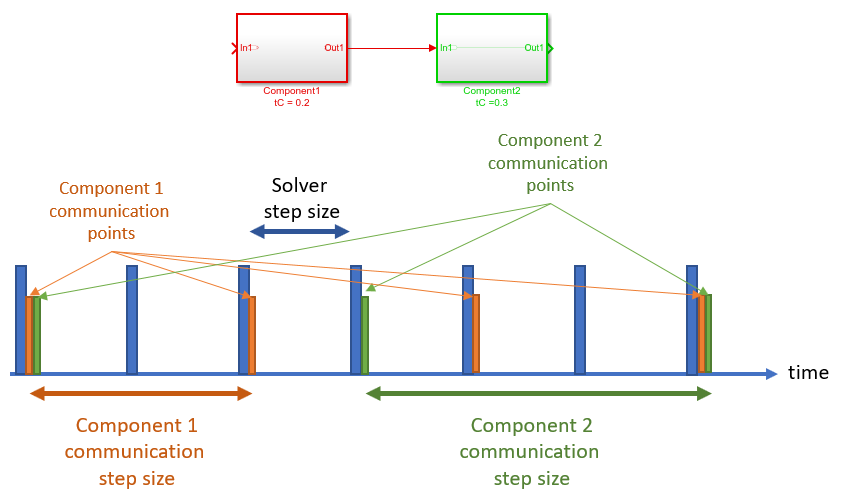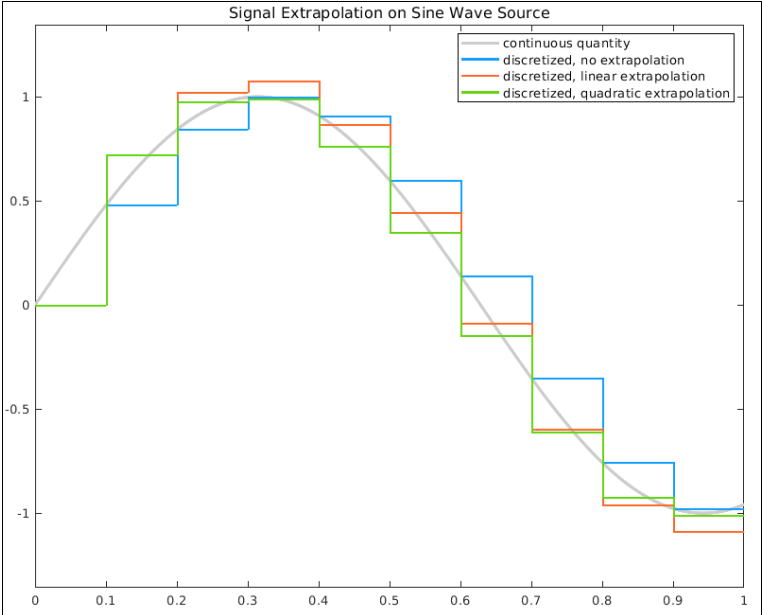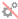Documentation

## Co-Simulation Execution and Numerical Compensation

Simulink® supports co-simulation between components with local solvers or involves external simulation tools. For example, co-simulation can involve an S-function implemented as a co-simulation gateway between Simulink and third-party tools or custom code. A co-simulation component can be a Functional Mockup Unit (FMU) in co-simulation mode imported to Simulink.

### Execution Timing

In Simulink simulation, the solver step size must be an integer divisor of each periodic, discrete block sample time. In other words, if a co-simulation component defines its own sample time, Simulink must communicate with the component at those time steps. Co-simulation can involve components whose time steps are determined internally and are not known to Simulink. The only information available to Simulink is the block sample time, either through the communication step size parameter of an FMU block, or the sample time definition in an S-function implementation. The block sample time determines the time steps Simulink must communicate with the co-simulation component. If the solver step size is not automatic, the communication step size must be an integer multiple of the solver step size.If the co-simulation component internally uses a local solver, then this local solver should also be taken into account when determining the communication step size of the block. The step size of the local solver is not exposed to Simulink, and knowledge of the implementation is necessary to set the communication step size correctly. The behavior of co-simulation in case of a potential incompatibility also depends on this internal solver implementation.

### Numerical Compensation

Co-simulation signals typically represent continuous physical quantities that are discretized due to co-simulation. Data exchanges between co-simulation components such as C MEX S-functions and Co-Simulation FMU blocks can introduce numerical inaccuracies from signal delays. Use numerical compensation to improve numerical behavior for simulation involving components that use their own solver. Model Advisor includes a check that detects co-simulation components and advises numerical compensation.

#### Numerical Compensation Prerequisites

Simulink automatically performs numerical compensation for co-simulation signals between co-simulation components. Simulink performs numerical compensation at the input of the destination block. A signal is automatically qualified for numerical compensation if its source port and destination port satisfy these conditions:

• The source port for the signal must satisfy these requirements:

• S-Function

• Output port data type is double

• Output port sample time is periodic and discrete

• Output port complexity is real

• `ssSetOutputPortIsContinuousQuantity()` is set to `true` for the port

• FMU

• Output port data type is double

• FMU is in co-simulation mode

• Block sample time is periodic and discrete

• Output port maps to a variable with `variability='continuous'` in `modelDescription.xml`

• The destination port for the signal must satisfy these requirements:

• S-Function

• Input port data type is double

• Input port sample time is periodic and discrete

• Input port complexity is real

• `ssSetInputPortIsContinuousQuantity()` is set to `true` for this port

• `ssSetInputPortDirectFeedThrough()` is set to `false` for this port

• FMU

• Input port data type is double

• FMU is in co-simulation mode

• Block sample time is periodic and discrete

• Input port maps to a variable with `variability='continuous'` in `modelDescription.xml`

For an example of identifying co-simulation signals for numerical compensation, see the `slexCoSimTripleMassSpringExample` model.

#### Manage Numerical Compensation Options Using the Dialog

When Simulink detects the signals that can have numerical compensation, it marks the corresponding input ports with theicon.

The following model includes co-simulation signals that can have numerical compensation:1. Open the model.

`slexCoSimTripleMassSpringExample`

2. Update the diagram. Simulink detects the signals that can have numerical compensation, and marks the corresponding ports with theicon.3. Adjust the parameters for compensation accuracy: Right-click the icon and select Configure Cosimulation Signal Compensation and adjust the calculation parameters:

• Extrapolation Method — This method computes a compensated signal value for the current time step of simulation using extrapolation of simulation signal values generated from previous time steps. Three types of extrapolations are offered for selection.

• `Linear` is the default, it uses signal values generated from previous two time steps to linearly estimate the signal value for use in the current time step of simulation.

• `Quadratic` uses signal values of the previous three time steps to fit the data to a quadratic polynomial.

• `Cubic` uses signal values of the previous four time steps to fit the data to a cubic polynomial.In the beginning of the simulation, when there is an insufficient number of past signal values, a lower order extrapolation method is used automatically. Higher order extrapolation methods use more past signal values to predict the current signal value and can improve accuracy of the prediction. However, high order extrapolation methods can also be numerically unstable. The best extrapolation method depends on the nature of the signal.

• Signal correction coefficient — This method further adjusts the extrapolated signal value based on past simulation results and past estimated signal values. A correction coefficient is offered for selection between `0` and `1`, where `0` means no adjustment to be made to the extrapolated signal value. The default setting for the correction coefficient is `1`. For a given extrapolated signal at a given time step, the larger the signal correction coefficient is, the more adjustment is made to the given extrapolated signal.

If numerical compensation is not beneficial, disable it by left-clicking theicon. When disabled, the icon appears with a red slash.

#### Manage Numerical Compensation Options from the Command Line

If automatic compensation is not possible, you can manually enable numerical compensation using the `CoSimSignalCompensationMode` property.

The `CoSimSignalCompensationMode` property has these values:

IconSettingBehavior`'Auto'`

Enable automatic numerical compensation, allowing Simulink to detect if the port has a signal eligible for numerical compensation.`'Auto_Off'`

Disable automatic numerical compensation. Icon appears with a red slash.`'Always'`

Force the port to be considered numerical compensation compliant, even if the signal is not eligible for numerical compensation. This setting lets you add compensation without declaring it to be continuous.`'Always_Off'`

Disable forcing the port to be considered numerical compensation compliant.

For example, to disable numerical compensation for the first input port from the previous model:

1. Select the block for which you want to select the port for numerical compensation. For example, get all the port handles for the currently selected block, `gcb`.

`p = get_param(gcb, 'PortHandles')`

This function returns all the ports for the currently selected block. For example,

```p = struct with fields: Inport: [22.0001 20.0001] Outport: [23.0001 25.0001] Enable: [] Trigger: [] State: [] LConn: [] RConn: [] Ifaction: [] Reset: []```
2. To disable numerical compensation for the first port:

```set_param(p.Inport(1), 'CoSimSignalCompensationMode', 'Auto_Off') ```

The associated port appears with a red slash.You can also set signal compensation parameters from the command line. The first step, again, is to obtain the port handles:

`p = get_param(block, 'PortHandles')`

Set the compensation parameters using the `CoSimSignalCompensationConfig` parameter, in this format:

`set_param(p.Inport,'CoSimSignalCompensationConfig','{<CompensationParam>:<ParamValue>}' )`

Find compensation parameter names and possible values in this table:

Compensation parameterParameter nameParameter value
`ExtrapolationMethod`Extrapolation method`'LinearExtrapolation'`, `'QuadraticExtrapolation'`, or `'CubicExtrapolation'`
`CompensationCoefficient`Compensation coefficientScalar between `0` and `1`

For example, set the extrapolation method for the port:

`set_param( p.Inport, 'CoSimSignalCompensationConfig', '{"ExtrapolationMethod":"LinearExtrapolation"}' ))`

Set both the extrapolation method and the compensation coefficient:

`set_param(p.Inport,'CoSimSignalCompensationConfig', '{"ExtrapolationMethod":"QuadraticExtrapolation", "CompensationCoefficient":"0.7"}' ))`

 Runge, Carl. "Uber empirische Funktionen und die Interpolation zwischen aquidistanten Ordinaten", Zeitschrift für Mathematik und Physik. Vol. 46, 1901, pp. 224–243.

Download now